# Distance Formula

Here we will learn about the distance formula, including how to find the distance between two coordinates.

There are also distance formula worksheets based on Edexcel, AQA and OCR exam questions, along with further guidance on where to go next if you’re still stuck.

## What is the distance formula?

The distance formula is an application of the Pythagorean theorem a^2+b^2=c^2 in coordinate geometry.

It will calculate the distance between two cartesian coordinates on an xy -coordinate plane.

To do this we find the differences between the x -coordinates and the difference between the y -coordinates, square them, then find the square root of the answer.

This can be written as the distance formula

d=\sqrt{\left(x_2-x_1\right)^2+\left(y_2-y_1\right)^2},

where d is the distance between the points \left(x_1, y_1\right) and \left(x_2, y_2\right) .

For example,

The line segment between the two points forms the hypotenuse of a right angled triangle.

The length of the hypotenuse is the distance between the two end points of the line segment.

### What is the distance formula?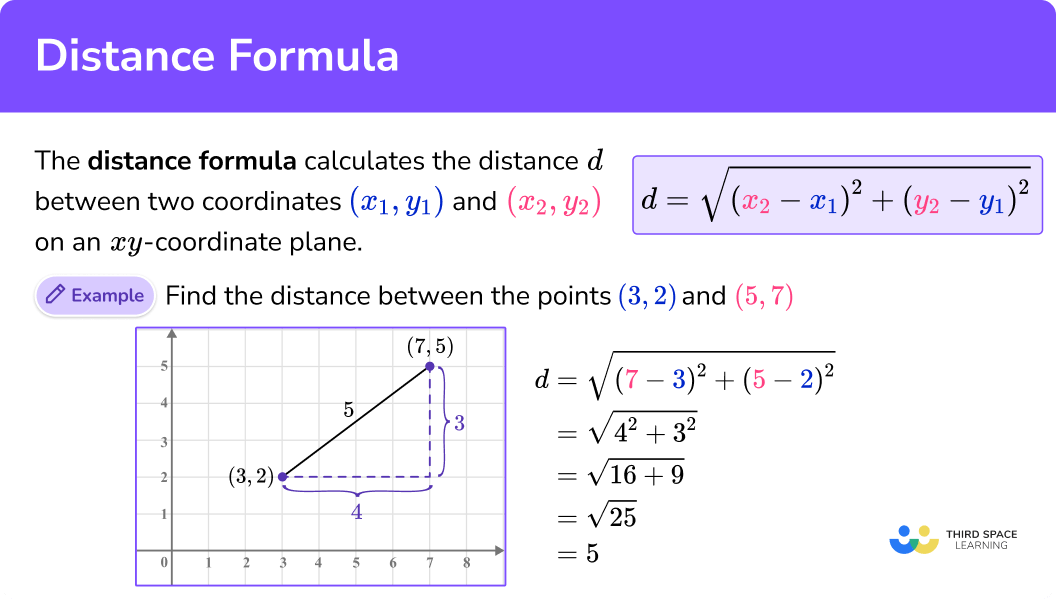## How to use the distance formula

In order to find the distance between two points:

1. Identify the two points and label them \bf{\left(x_1, y_1\right) } and \bf{\left(x_2, y_2\right)} .
2. Substitute the values into the formula \bf{d=\sqrt{\left(x_2-x_1\right)^2+\left(y_2-y_1\right)^2}} .
3. Solve the equation.

### Explain how to use the distance formula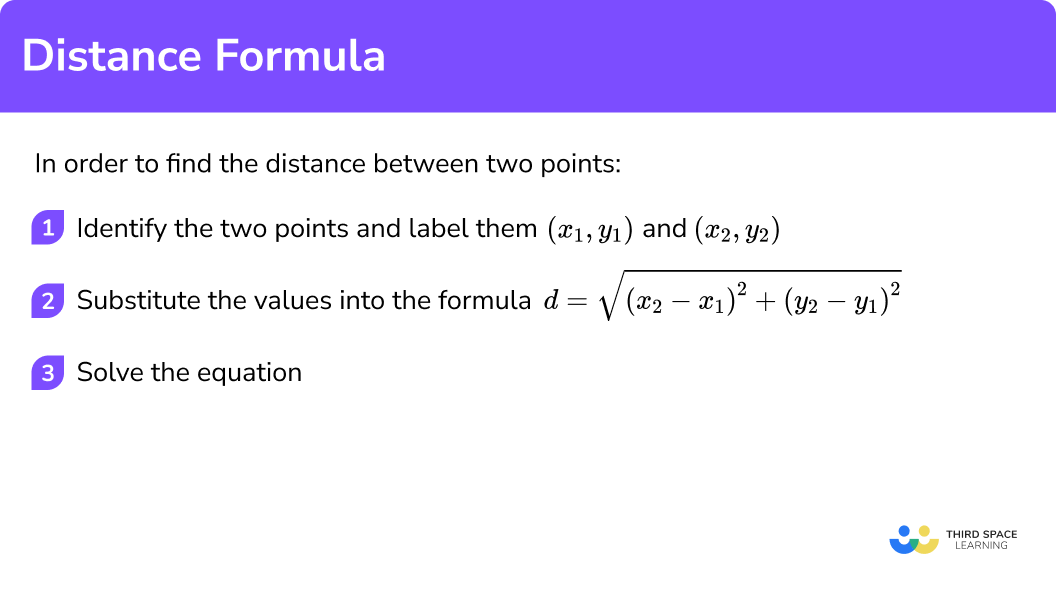### Related lessons on straight line graphs

Distance formula is part of our series of lessons to support revision on straight line graphs. You may find it helpful to start with the main straight line graphs lesson for a summary of what to expect, or use the step by step guides below for further detail on individual topics. Other lessons in this series include:

## Distance formula examples

### Example 1: distance between two points on a coordinate axes in the first quadrant

Find the distance between the points A and B.

1. Identify the two points and label them \bf{\left(x_1, y_1\right)} and \bf{\left(x_2, y_2\right)} .

A=(3,1) and B=(6, 5).

Let \left(x_1, y_1\right) =(3, 1) and \left(x_2, y_2\right) =(6, 5).

2Substitute the values into the formula \bf{d=\sqrt{\left(x_2-x_1\right)^2+\left(y_2-y_1\right)^2}} .

\begin{aligned} d&=\sqrt{\left(x_2-x_1\right)^2+\left(y_2-y_1\right)^2} \\\\ &=\sqrt{(6-3)^2+(5-1)^2} \end{aligned}

3Solve the equation.

\begin{aligned} d&=\sqrt{(6-3)^2+(5-1)^2} \\\\ &=\sqrt{3^2+4^2} \\\\ &=\sqrt{9+16} \\\\ &=\sqrt{25} \\\\ &=5 \end{aligned}

### Example 2: distance between two points on a coordinate axes

Find the distance between the points A and B.

Identify the two points and label them \bf{\left(x_1, y_1\right)} and \bf{\left(x_2, y_2\right)} .

Substitute the values into the formula \bf{d=\sqrt{\left(x_2-x_1\right)^2+\left(y_2-y_1\right)^2}} .

Solve the equation.

### Example 3: distance between two given points with positive coordinates

Find the distance between the points (1, 4) and (7, 12).

Identify the two points and label them \bf{\left(x_1, y_1\right)} and \bf{\left(x_2, y_2\right)} .

Substitute the values into the formula \bf{d=\sqrt{\left(x_2-x_1\right)^2+\left(y_2-y_1\right)^2}} .

Solve the equation.

### Example 4: distance between any two given points

Find the distance between the points (-2, 5) and (6, -7).

Identify the two points and label them \bf{\left(x_1, y_1\right)} and \bf{\left(x_2, y_2\right)} .

Substitute the values into the formula \bf{d=\sqrt{\left(x_2-x_1\right)^2+\left(y_2-y_1\right)^2}} .

Solve the equation.

### Example 5: finding a missing value given the distance

The distance between the points (1, 5) and (16, \, k) is 17.

Find the value of k, where k is negative.

Identify the two points and label them \bf{\left(x_1, y_1\right)} and \bf{\left(x_2, y_2\right)} .

Substitute the values into the formula \bf{d=\sqrt{\left(x_2-x_1\right)^2+\left(y_2-y_1\right)^2}} .

Solve the equation.

### Common misconceptions

• Confusing the distance formula with the midpoint formula

A common error is to find the midpoint instead of the distance.

The midpoint formula is \left(\cfrac{x_1+x_2}{2}, \, \cfrac{y_1+y_2}{2}\right).

• Squaring negative numbers to give a negative

When using the distance formula, it is common to get negative values after the subtraction step. These values will be squared, so it is important to remember that the square of a negative value is positive. For example, (-3)^2=9.

### Practice distance formula questions

1. Find the distance between the point (6, 8) and the origin.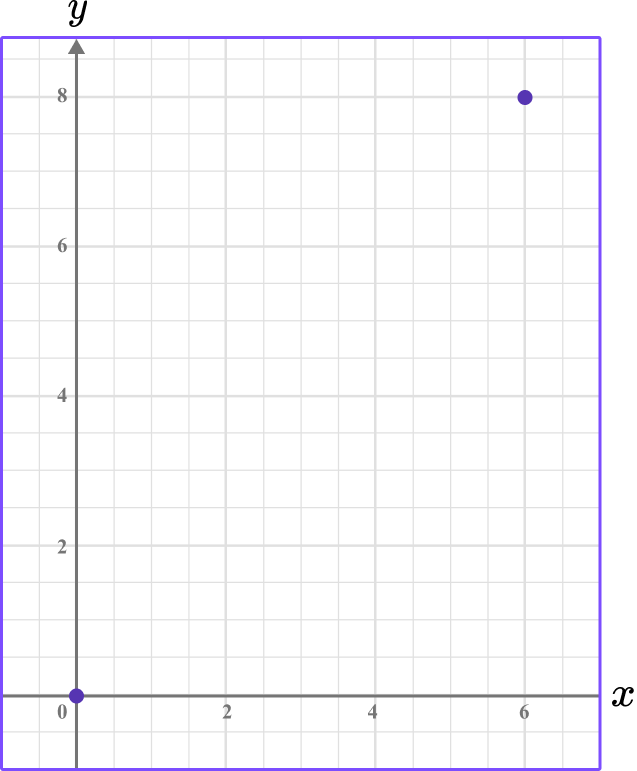14103.74100The origin is (0, 0) so let \left(x_1, y_1\right)=(0, 0) and \left(x_2, y_2\right)=(6, 8).

\begin{aligned} d&=\sqrt{\left(x_2-x_1\right)^2+\left(y_2-y_1\right)^2} \\\\ & =\sqrt{(6-0)^2+(8-0)^2} \\\\ & =\sqrt{6^2+8^2} \\\\ & =\sqrt{36+64} \\\\ & =\sqrt{100} \\\\ & =10 \end{aligned}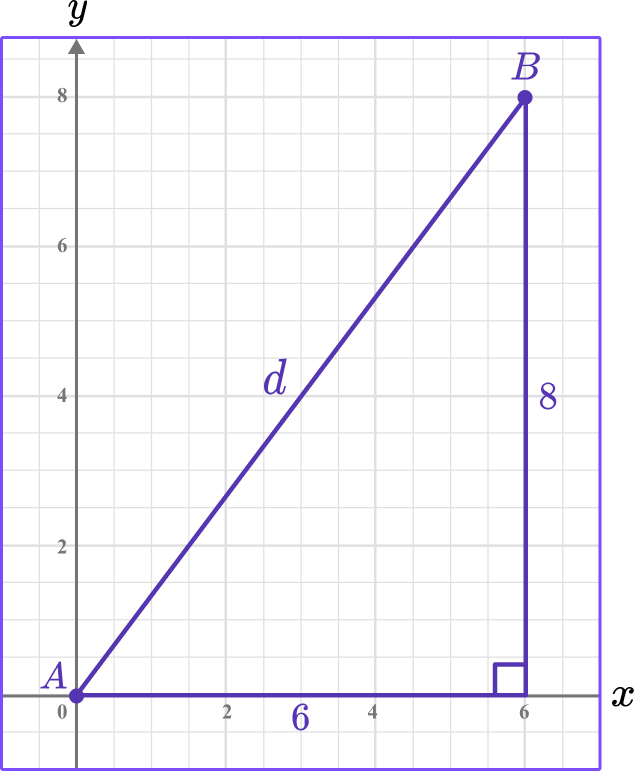2. Find the distance between the point (0, 10) and (24, 0).

345.832614Let \left(x_1, y_1\right)=(0, 10) and \left(x_2, y_2\right)=(24, 0).

\begin{aligned} d&=\sqrt{\left(x_2-x_1\right)^2+\left(y_2-y_1\right)^2} \\\\ & =\sqrt{(24-0)^2+(0-10)^2} \\\\ & =\sqrt{24^2+(-10)^2} \\\\ & =\sqrt{576+100} \\\\ & =\sqrt{676} \\\\ & =26 \end{aligned}

3. Find the distance between the points (5, 3) and (14, 10). Give your answer to 1 decimal place.

11.513023.011.4Let \left(x_1, y_1\right)=(5, 3) and \left(x_2, y_2\right)=(14, 10).

\begin{aligned} d&=\sqrt{\left(x_2-x_1\right)^2+\left(y_2-y_1\right)^2} \\\\ & =\sqrt{(14-5)^2+(10-3)^2} \\\\ & =\sqrt{9^2+7^2} \\\\ & =\sqrt{81+49} \\\\ & =\sqrt{130} \\\\ & =11.4 \text { (1 d.p.) } \end{aligned}

4. Find the distance between the points (-2, 4) and (-8, -9). Give your answer to 1 decimal place.

14.31916.47.8Let \left(x_1, y_1\right)=(-2, 4) and \left(x_2, y_2\right)=(-8, -9).

\begin{aligned} d&=\sqrt{\left(x_2-x_1\right)^2+\left(y_2-y_1\right)^2} \\\\ & =\sqrt{(-8-(-2))^2+(-9-4)^2} \\\\ & =\sqrt{(-6)^2+(-13)^2} \\\\ & =\sqrt{36+169} \\\\ & =\sqrt{205} \\\\ & =14.3 \text { (1 d.p.) } \end{aligned}

5. The distance between the points (8,-3) and (15, a) is 25. Find the value of a, where a is positive.

-272127-21\begin{aligned} d&=\sqrt{\left(x_2-x_1\right)^2+\left(y_2-y_1\right)^2} \\\\ 25&=\sqrt{(15-8)^2+(a-(-3))^2} \\\\ 25&=\sqrt{7^2+(a+3)^2} \\\\ 625&=49+(a+3)^2 \\\\ 576&=(a+3)^2 \\\\ \pm 24&=a+3 \\\\ a&=\pm 24-3 \end{aligned}

a=21 or a=-27

We know that a is positive so a=21.

6. The distance between the points (b, 4) and (6, -8) is 15. Find the value of b, where b is negative.

3-15-315\begin{aligned} d&=\sqrt{\left(x_2-x_1\right)^2+\left(y_2-y_1\right)^2} \\\\ 15&=\sqrt{(6-b)^2+(-8-4)^2} \\\\ 15&=\sqrt{(6-b)^2+(-12)^2} \\\\ 225&=(6-b)^2+144 \\\\ 81&=(6-b)^2 \\\\ \pm 9&=6-b \\\\ -b&=\pm 9-6 \end{aligned}

-b=3 or -b=-15

b=-3 or b=15

We know that b is negative so b=-3.

### Distance formula GCSE questions

1. Find the distance between (0, 4) and (7, 18).

(3 marks)

7^2+14^2

(1)

\sqrt{245}

(1)

Answer in the range of 15.6-15.7.

(1)

2.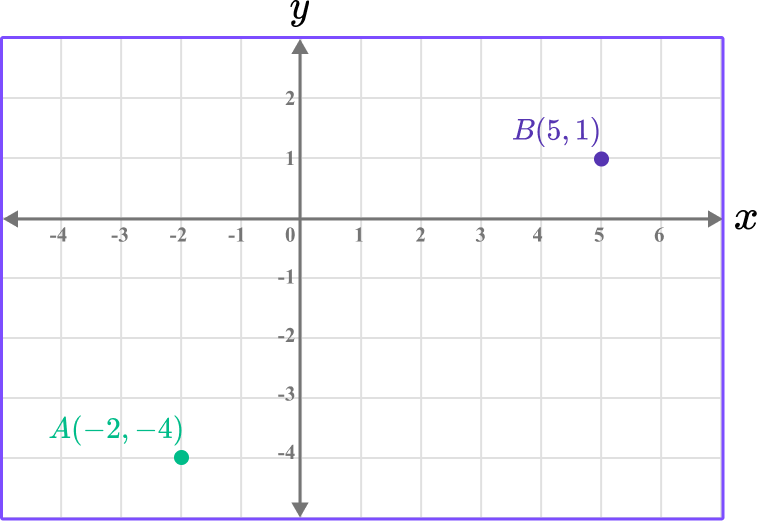(a) Find the midpoint of AB.

(b) Find the distance AB.

(5 marks)

(a)

x -value of 1.5.

(1)

y -value of -1.5.

(1)

(b)

7^2+5^2

(1)

\sqrt{74}

(1)

Answer in the range of 8.6-8.7.

(1)

3. M(4,3) is the midpoint of the line segment PQ, where Q has the coordinates (-9, 7).

Find the distance PQ to one decimal place.

(4 marks)

Process to find the point P \, (17,-1).

(1)

(17-(-9))=26 \, or \, (-1-7)=-8

(1)

26^2+(-8)^2=740

(1)

27.2-27.3

(1)

Alternatively, find the distance MQ and double it.

(4-(-9))=13 \, or \, (3-7)=-4

(1)

13^2+4^2=185

(1)

2\times \sqrt{185}

(1)

27.2-27.3

(1)

## Learning checklist

You have now learned how to:

• Find the distance between two points on an xy -coordinate axes

## Beyond GCSE

In A level mathematics, we look at coordinates in three dimensional space. The 2D distance formula can be adapted for a 3D coordinate system that has an x -axis, y -axis and z -axis with coordinates \left(x_1, y_1, z_1\right) and \left(x_2, y_2, z_2\right) .

d=\sqrt{\left(x_2-x_1\right)^2+\left(y_2-y_1\right)^2+\left(z_2-z_1\right)^2}

## Still stuck?

Prepare your KS4 students for maths GCSEs success with Third Space Learning. Weekly online one to one GCSE maths revision lessons delivered by expert maths tutors.

Find out more about our GCSE maths tuition programme.